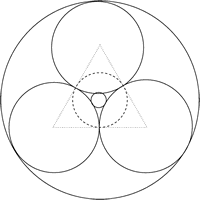You may also likeBaby Circle

A small circle fits between two touching circles so that all three circles touch each other and have a common tangent? What is the exact radius of the smallest circle?Kissing

Two perpendicular lines are tangential to two identical circles that touch. What is the largest circle that can be placed in between the two lines and the two circles and how would you construct it?Logosquares

Ten squares form regular rings either with adjacent or opposite vertices touching. Calculate the inner and outer radii of the rings that surround the squares.

Circles in Circles

Age 16 to 18Challenge Level

Take three unit circles, each touching the other two. Construct three circles $C_1$, $C_2$ and $C_3$, with radii $r_1$, $r_2$ and $r_3$, respectively, as in the figure below. The circles that are tangent to all three unit circles are $C_1$ and $C_3$, with $C_1$ the smaller of these. The circle through the three points of tangency of the unit circles is $C_2$. Find the radii $r_1$, $r_2$ and $r_3$, and show that $r_1r_3=r_2^2$.